# Terminal Velocity of a Human, Free Fall and Drag Force

Eugene is a qualified control/instrumentation engineer Bsc (Eng) and has worked as a developer of electronics & software for SCADA systems.

## What Happens to an Object That's Falling in a Vacuum?

We all know that when an object is released from a certain height, it starts to fall. This, of course, is due to gravity, or more specifically the gravitational force of attraction between the object and the Earth. The force of gravity causes the object to accelerate and increase in velocity as it falls downwards towards the Earth. In actuality, both the Earth and the object are mutually attracted to each other, and the Earth moves upwards at the same time. However, since it's so massive in comparison to a small object and the force is so small, its movement is minuscule.

## Definitions of Quantities Used in Kinematics

Before we go any further, let's define some of the terms used in kinematics, which is an area of physics concerned with the motion of objects.

• Mass: the amount of matter in an object. The greater the mass of an object, the greater the amount of inertia it has and its reluctance to move.
• Speed: the rate of change of position of an object (how fast something moves).
• Velocity: speed in a given direction. Velocity is a vector quantity, which means it has a magnitude called speed and also a direction. In physics, we generally talk about velocity rather than speed.
• Force: a push or pull. A force causes an object with mass to accelerate.
• Acceleration: the rate at which velocity changes.
• Free Fall: when an object falls under the influence of gravity alone without other forces acting on it.

See my beginner's guide to mechanics for a more detailed understanding of the basics of forces and motion:

Newton's Laws of Motion and Understanding Force, Mass, Acceleration, Velocity, Friction, Power and Vectors

## Does Velocity Keep Increasing When Something Falls?

If an object falls in a vacuum outside Earth's atmosphere, its velocity continues to increase because of the acceleration due to gravity. This is called free fall. However, if the object falls through the air (or another fluid such as water), this limits the maximum velocity it can reach.The force of gravity causes objects to accelerate.© Eugene Brennan

## Drag Force

When an object moves through a fluid, it experiences a force which opposes motion and tends to slow it down. This force is called drag. The fluid could be a liquid, such as water, or a mixture of gasses, such as air. If you put your hand out the window of a moving car or try to wade through water, you can feel this force.

### What Affects Drag?

Drag increases on an object as it moves faster. In fact, it increases exponentially, which means if velocity doubles, drag increases four times and if velocity triples, drag goes up nine times and so on. Drag is also influenced by the shape or surface geometry of an object. Aircraft, trains, ships, road vehicles, bullets and missiles are streamlined to minimise drag, reduce energy consumption and decrease in velocity.

When an object is dropped in a vacuum, it free falls, acted on by gravity alone. However, if it is dropped within Earth's atmosphere, it experiences drag which slows it down.

The force of gravity acts downwards, and the drag force acts upwards.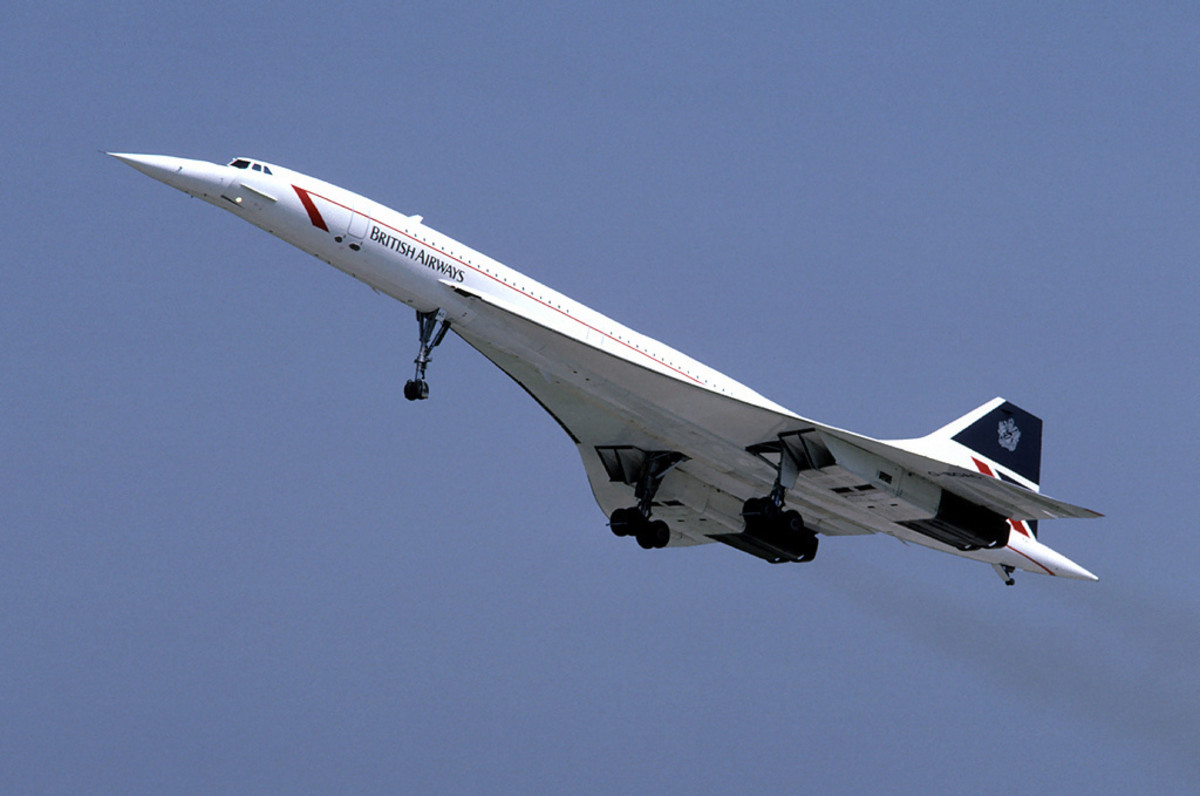The supersonic passenger aircraft Concorde. Aircraft have slim, streamlined profiles to reduce drag.Eduard Marmet, CC BY SA 2.0 Unported via Wikimedia Commons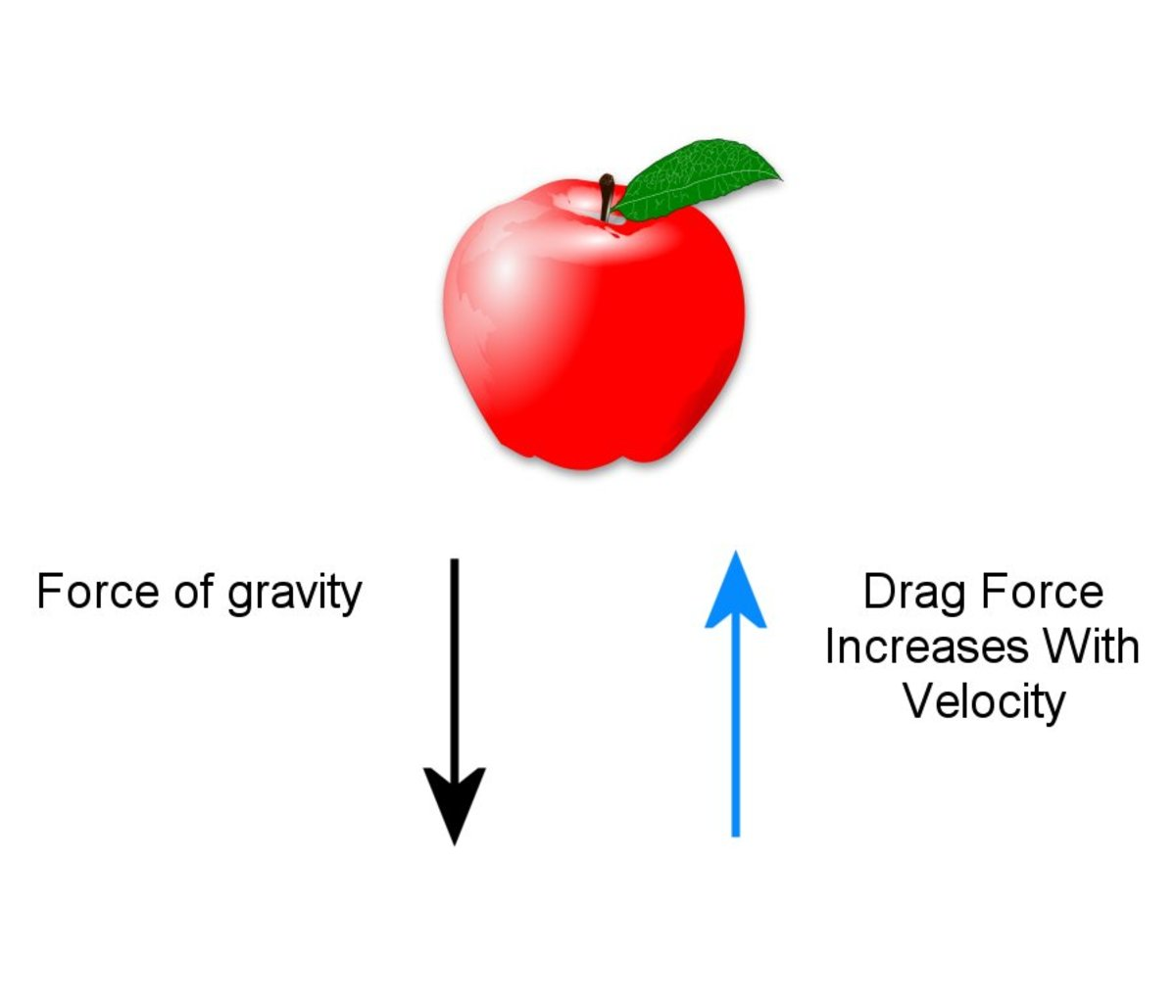A force called drag opposes the force of gravity.© Eugene Brennan
Scroll to Continue

## What Is Weight?

Mass is the amount of matter in a body, but in physics, mass and weight have very specific meanings. While the mass of an object is the same, irrespective of where it is located in the Universe, weight varies. Weight is the gravitational force between objects and equals mass multiplied by the acceleration due to gravity g.

So the force of gravity or weight is

Fg = mg

Where Fg is the force measured in Newtons (N)

m is the mass of an object in kilograms (kg)

and g is the acceleration due to gravity in metres per second squared (m/s2)

g is approximately 9.81 metres per second per second, written as 9.81 m/s/s or m/s2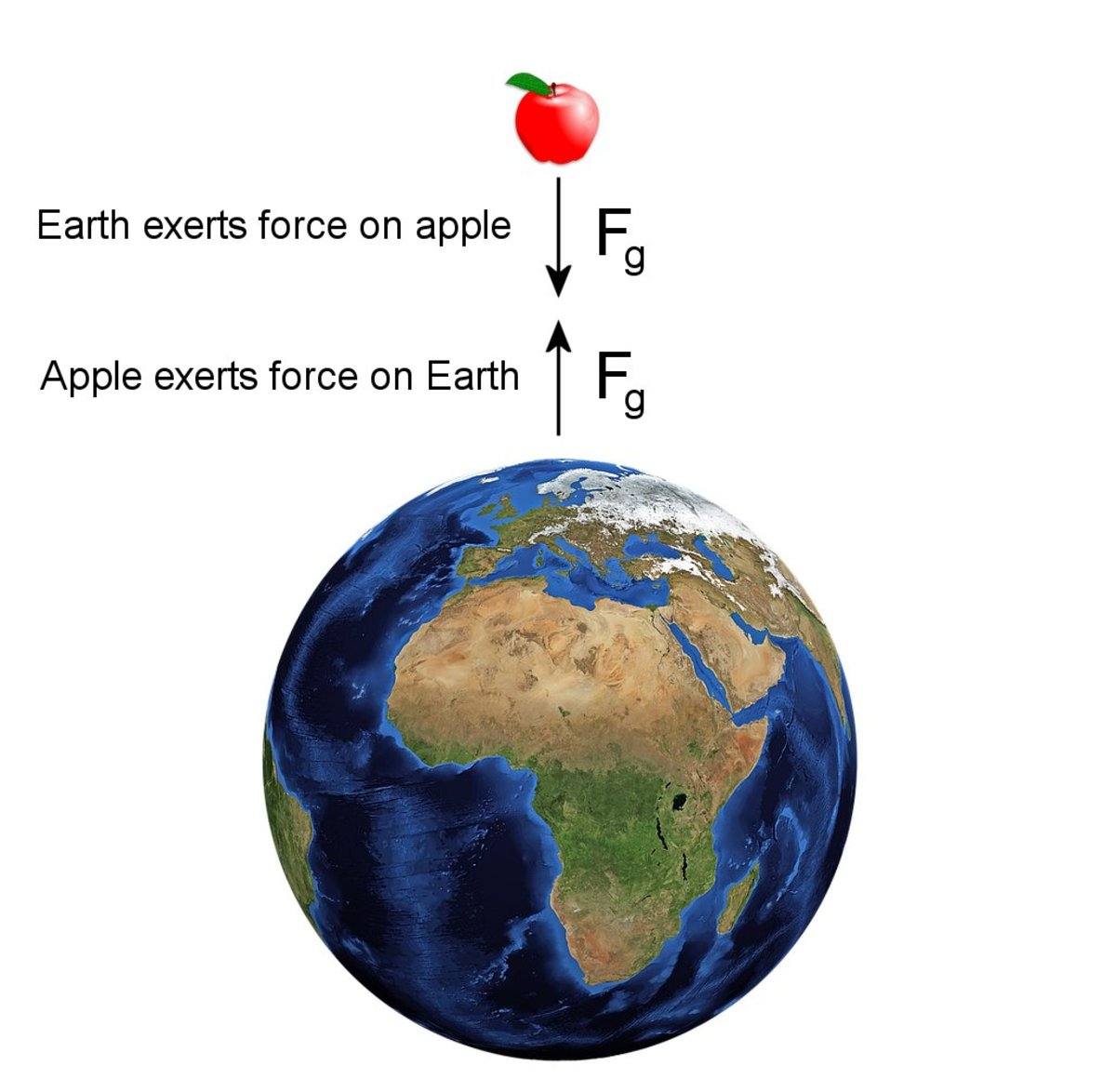There is a mutual attraction between Earth and other objects.© Eugene Brennan

## At Equilibrium, Drag Force Equals Weight of the Object

The net force acting on a free-falling body is the difference between the weight acting down and the drag force acting upwards. As long as this is positive, the body keeps accelerating downwards.

Since the drag force increases with velocity, eventually at some stage it equals the weight of the falling body (which isn't changing and stays constant at Fg = mg).
Once this equilibrium point is reached, since the two forces are equal, there is no net force on the object. No net force means no more force to keep accelerating the body, so its velocity reaches a maximum known as the terminal velocity.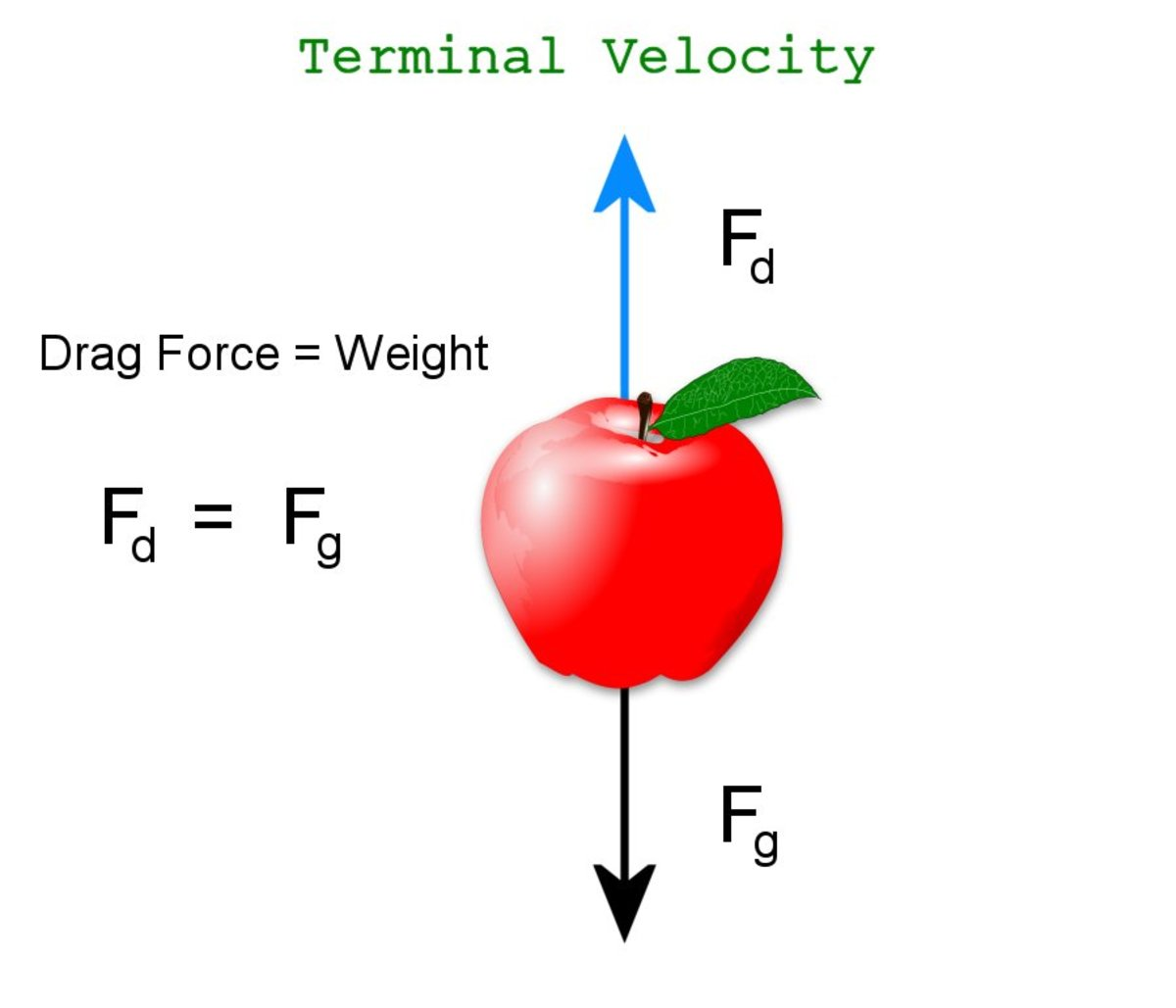As velocity increases, the drag force acting upwards eventually equals the force of gravity acting downwards, the net force becomes zero and an object no longer accelerates. It has reached terminal velocity.© Eugene Brennan

## Velocity of a Falling Object With No Drag

As an aside, let's look at the equation for the velocity of a falling object when there's no drag. If an object falls through a vacuum without being slowed down by a drag force, its velocity v in m/s is given by the equation:

v = √(2gh)

where g is the acceleration due to gravity.

and h is the distance fallen in metres (m)

In terms of time t in seconds since the object was dropped, another equation for velocity is:

v = gt

To put this into perspective, after 10 seconds of free fall in a vacuum, an object would be traveling at:

v = gt = 9.81 x 10 = 98.1 m/s or 355 km/hr (219 miles per hour)

However, as we shall see, drag puts an upper limit on velocity.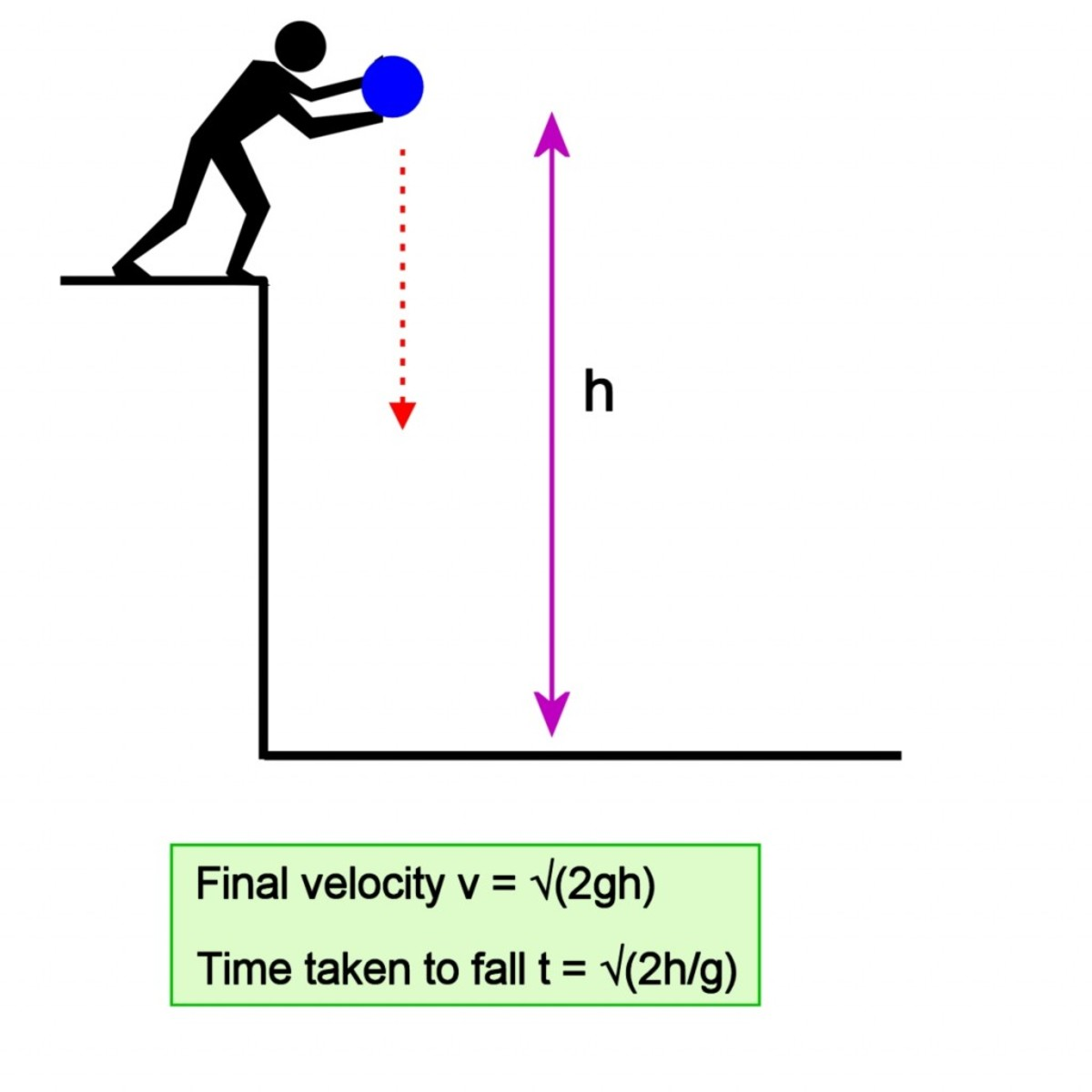Without an atmosphere and drag, falling objects would increase in velocity until they hit the ground.© Eugene Brennan

## The Drag Equation

The drag equation describes the force experienced by an object moving through a fluid:

If Fd is the drag force, then:

Fd = ½ ρ u2 Cd A

Where Fd is the force in newtons (N)

p is the density of the fluid in kilograms per cubic metre (kg/m3)

u is the velocity of the object relative to the fluid in metres per second (m/s)

Cd is the drag coefficient that depends on the shape of the object and the nature of its surface

and A is the area of the orthogonal projection of the object in m2. This can be visualized as the area of the shadow of the object cast on a surface if a light with a parallel beam was shone on it and landed perpendicular to the surface.
Because of the u2 term in the equation, drag increases with the square of the velocity.

## Derivation of Terminal Velocity

At equilibrium, the drag force Fd acting upwards equals the weight Fg
acting downwards

We know Fd = ½ ρ u2 Cd A

and Fg = mg

At equilibrium, the velocity becomes the terminal velocity. Let's call it Vt

Equate Fg to Fd and replace u by Vt giving:

mg = ½ ρ u2 Cd A = ½ ρ Vt2 Cd A

So:

2mg = ρ Vt2 Cd A

Divide both sides by ρ Cd A giving:

2mg / ρ Cd A = Vt2

Taking the square root of both sides gives us:

Vt = √((2mg) / (ρACd)

## Terminal Velocity of a Human

From the equation for terminal velocity, we see it depends on several factors:

• Density of the air.
• Mass of the object
• Area of the object
• Acceleration due to gravity (this doesn't really change, so it can be assumed to be practically constant)
• The shape of the object

For a human, the drag coefficient Cd is about 1 in a belly down, horizontal orientation and 0.7 in head down position.

Typically in this position, terminal velocity is about 120 mph or 54 m/s.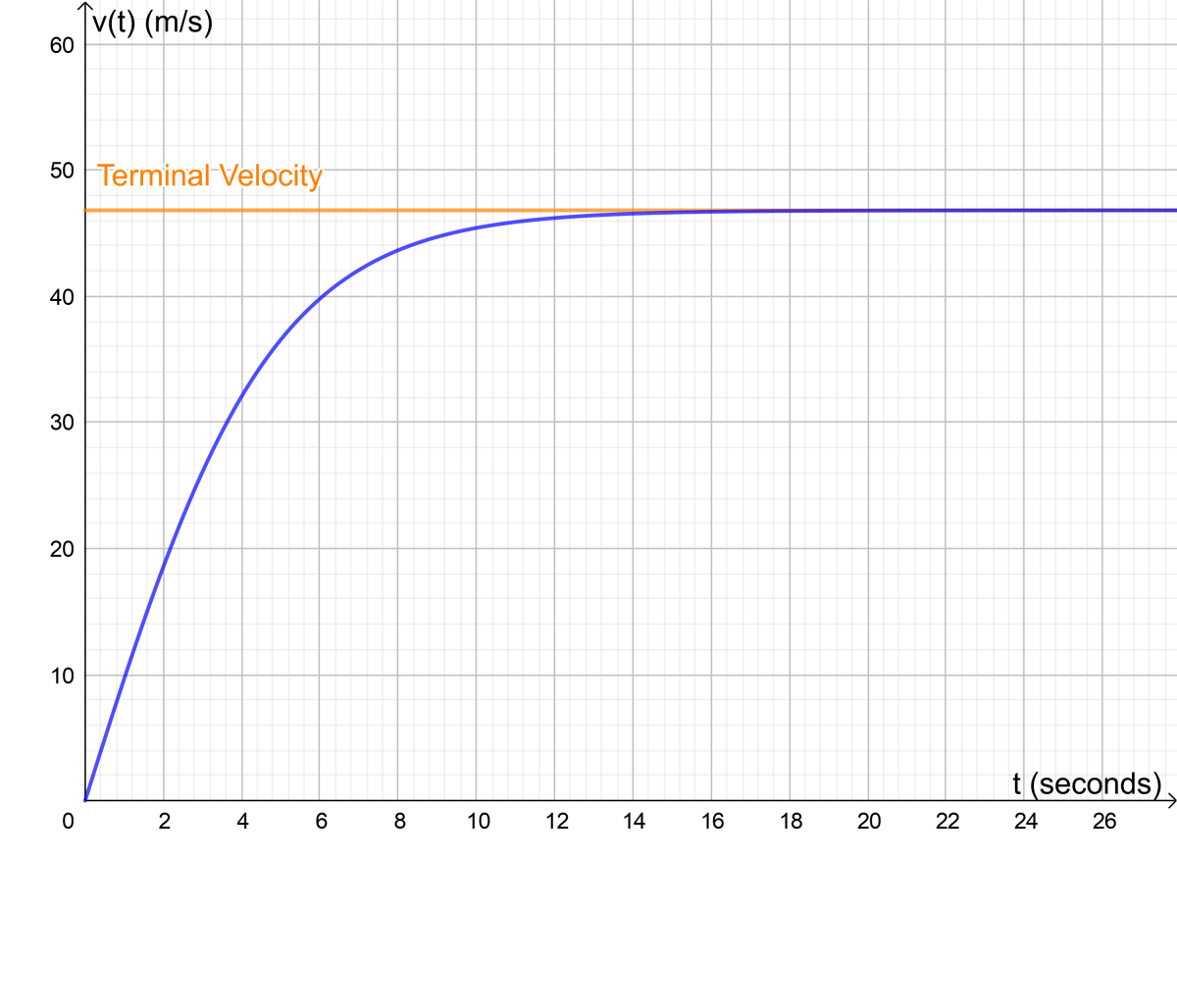Instantaneous and terminal velocity for a 100kg, 1.8m tall human lying horizontally. Terminal velocity is reached after about 14 seconds.© Eugene Brennan

## How Long Does It Take to Reach Terminal Velocity and How Far Does a Human Fall?

It takes about 12 seconds to reach 97% of terminal velocity. During that period, a human would fall about 455 metres.

## What Increases Terminal Velocity?

Speed skydivers compete by trying to reach the highest possible terminal velocity. From the equation, we can see that it can be increased by:

• being heavier
• diving in thinner, low density air
• reducing the projected area by diving head first
• reducing the drag coefficient by diving head first.
• wearing clothing that improves streamlining and reduces drag